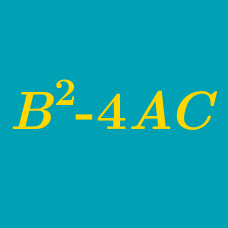Algebra

# Quadratic Discriminant - Problem Solving

If $a, b, c$ are real numbers such that $c (a+b+ c ) < 0$, what can we say about $b^2 - 4ac$?

Hint: Think about the function $f(x) = ax^2 + bx + c$.

For what values of $m$ are there no intersection points between the line $y= mx + 13$ and the circle $x^2 + y^2 = 17$?

$k$ is uniformly chosen from the interval $[ -5, 5]$. Let $p$ be the probability that the quadratic $f(x) = x^2 + kx + 1$ has both roots between -2 and 4. What is the value of $\lfloor 1000 p \rfloor$?

Details and assumptions

Greatest Integer Function: $\lfloor x \rfloor: \mathbb{R} \rightarrow \mathbb{Z}$ refers to the greatest integer less than or equal to $x$. For example $\lfloor 2.3 \rfloor = 2$ and $\lfloor -3.4 \rfloor = -4$.

For how many positive integer values of c does the equation $2x^2 + 705x +c = 0$ have an integer solution?

For a positive integer $m$ such that $m \le 25,$ what is the probability that the quadratic equation $x^2+mx+\frac{1+m}{2}=0$ has real roots?

×